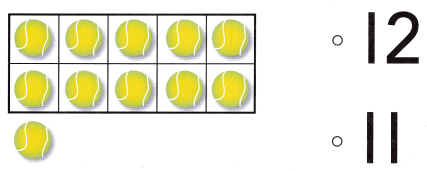# Texas Go Math Kindergarten Lesson 7.1 Answer Key Model and Count 11 and 12

Refer to our Texas Go Math Kindergarten Answer Key Pdf to score good marks in the exams. Test yourself by practicing the problems from Texas Go Math Kindergarten Lesson 7.1 Answer Key Model and Count 11 and 12.

## Texas Go Math Kindergarten Lesson 7.1 Answer Key Model and Count 11 and 12

Explore

DIRECTIONS: Use counters to show the number 11. Add more to show the number 12. Draw the counters.Explanation:
One added to 11 = 11 + 1 = 12.

Share and Show

DIRECTIONS: 1. Count and tell how many. Circle the number and word. 2. Use counters to show the number 11. Draw the counters. 3. Look at the counters you drew. How many are in the ten frame? Write the number. How many more are there? Write the number.

Question 1.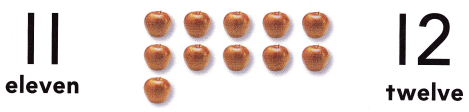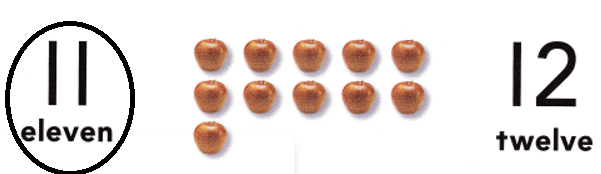Explanation:
Number of apples = 11 or Eleven.

Question 2.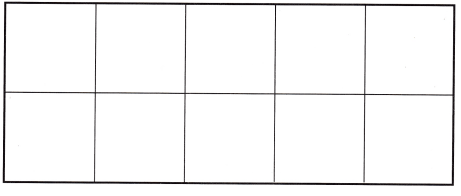Explanation:
Counters = 10 + 1 = 11.

Question 3.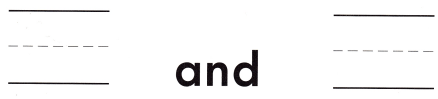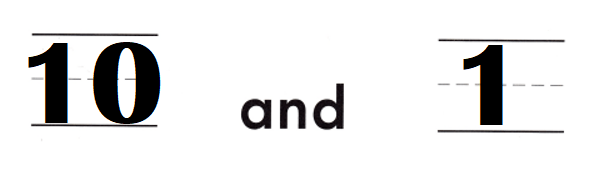Explanation:
Number of counters in frame = 10.
Number of counters out of frame = 1
Total counters = Number of counters in frame + Number of counters out of frame
= 10 + 1
= 11.

DIRECTIONS: 4. Count and tell how many. Circle the number and word. 5. Use counters to show the number 12. Draw the counters. 6. Look at the counters you drew. How many are in the ten frame? Write the number. How many more ore there? Write the number.

Question 4.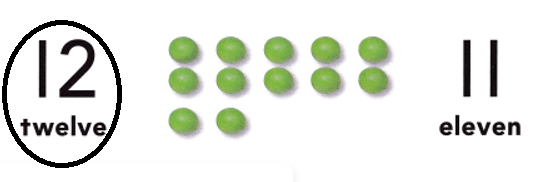Explanation:
Number of dots = 12 or Twelve.

Question 5.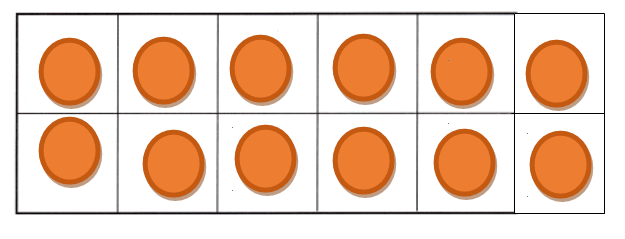Explanation:
Number of counters = 12 or Twelve.

Question 6.Explanation:
Number of dots in frame = 10
Number of dots out of frame = 2.
Total dots = Number of dots in frame + Number of dots out of frame
= 10 + 2
= 12.

HOME ACTIVITY • Draw a ten frame on a sheet of paper. Have your child use small objects such as buttons, pennies, or dried beans to show the numbers 11 and 12.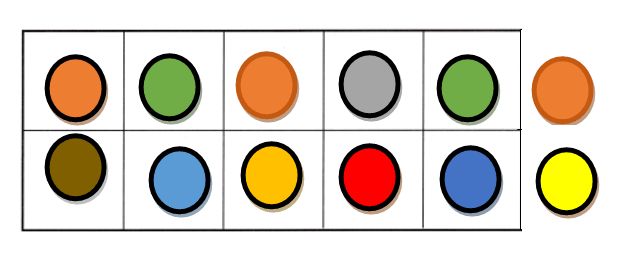Explanation:
My kid took different types of buttons and placed them in the frame and out of the frame to give the count 12 = 10 + 2.

Problem Solving

Question 7.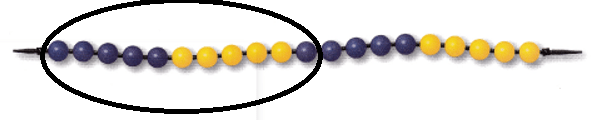Explanation:
Number of blue beads = 6.
Number of yellow beads = 5.
= 6 + 5
= 11.

Question 8.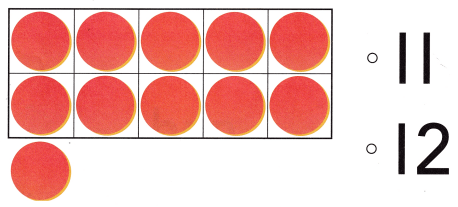Explanation:
Number of counter = 10 + 1 = 11.

### Texas Go Math Kindergarten Lesson 7.1 Homework and Practice Answer Key

DIRECTIONS: 1. Draw counters to show 11. Write the number. 2. Draw counters to show 12. Write the number.

Question 1.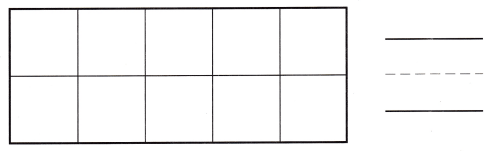Explanation:
Number of counters = 10 + 1 = 11.

Question 2.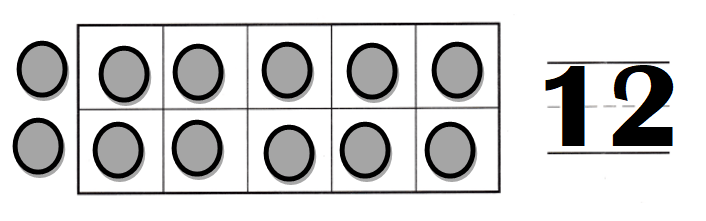Explanation:
Number of counters = 10 + 2 = 12.

DIRECTIONS: Choose the correct answer. 3. How many bears are there? 4. How many cars are there? 5. How many balls are there?

Lesson Check

Question 3.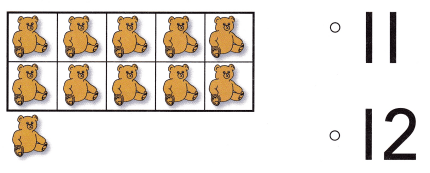Explanation:
Number of bears = 10 + 1 = 11 or Eleven.

Question 4.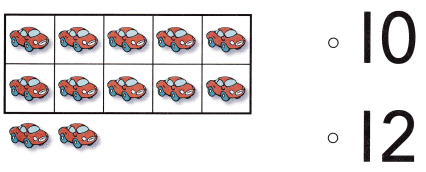Explanation:
Number of cars = 12 or Twelve.

Question 5.